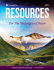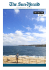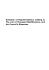# Chapter 3

## Transcription

Chapter 3
```CHAPTER 3
Convergence and Approximation Theorems
3.1. Compact convergence
Let us begin by introducing a notion of convergence which plays a central role
in complex function theory. Our primary interest in this chapter is convergence
in the space of holomorphic functions in an open subset of the complex plane.
However, it will be convenient to consider convergence in the space of continuous
maps between two metric spaces. There are at least two motivations behind this more
general approach: First, the arguments in the general case are virtually identical to
the special case, so one can treat all the important cases in one stroke at no extra
cost. More importantly, this approach allows us to apply the convergence machinery
to holomorphic maps between Riemann surfaces (in particular, to meromorphic
functions) which will be studied in chapter 9.
In what follows dX will denote the distance function associated with a metric
space X .
Definition 3.1. Let X and Y be metric spaces. We say that a sequence of
maps fn W X ! Y converges compactly to f W X ! Y as n ! 1 if
for every compact set K X , the sequence of restrictions fn jK converges
uniformly to f jK as n ! 1. In other words, if for every compact set K X
and every " > 0 there exists an integer N 1 such that
sup dY .fn .p/; f .p// < "
whenever n N:
p2K
The phrase uniform convergence on compact subsets is also widely used in this
context.
Recall that a metric space X is locally compact if each point in X has an open
neighborhood with compact closure and is called -compact if X is a union of
countably many compact subsets. Evidently these conditions hold for our main
examples in this chapter, namely when X is an open subset of the plane.
One should have no difficulty verifying the following result:
82
3 Convergence and Approximation Theorems
Theorem 3.2.
(i) Suppose fn ! f compactly in X and each fn is continuous. Then f
is continuous.
(ii) Suppose X is locally compact. Then fn ! f compactly in X if and
only if every point in X has a neighborhood U such that fn ! f
uniformly in U .
Because of part (ii) of this theorem, in the locally compact case (such as open
subsets of the plane), compact convergence is also referred to as local uniform
convergence.
P
Pn
k
k
Example 3.3. Let f .z/ D 1
kD0 ak z be holomorphic in D. Define fn .z/ D
kD0 ak z .
Then fn ! f compactly in D as n ! 1 (Theorem 1.17). However, the convergence is
not necessarily uniform in D. For example, when f .z/ D 1=.1 z/, we have fn .z/ D
.1 z nC1 /=.1 z/ and so
ˇ nC1 ˇ
ˇ
ˇz
ˇ D C1:
sup jfn .z/ f .z/j D sup ˇˇ
1 zˇ
z2D
z2D
Let Map.X; Y / denote the space of all continuous maps X ! Y . Assuming
X is locally compact and -compact, we can equip the space Map.X; Y / with a
metric d with the property that d.fn ; f / ! 0 if and only if fn ! f compactly in
X . To see this, write X as the union of the countable collection of compact subsets
fEn g. Set K1 D E1 . Since X is locally compact, the compact set K1 [ E2 can be
covered by finitely many open sets U1 ; : : : ; Um such that each Uk is compact. Then
K2 D U1 [ [ Um is compact and contains both K1 and E2 in its interior. Repeating
the same argument for K2 [ E3 , we obtain a compact set K3 which contains both K2
and E3 in its interior. Continuing inductively, we obtain a sequence fKn g of compact
subsets of X such that
S
XD 1
nD1 Kn , and
Lemma 3.32 will give
exhaustions with an
property that will be
needed in Runge’s theory.
That property is not
needed here.
each Kn is contained in the interior of KnC1 .
We call such a sequence fKn g an exhaustion of X . The most useful property here
is that every compact set K X is contained in some Kn . In fact, K is covered by
the union of the interiors of the Kn , and since these interiors are nested, one of them
Given an exhaustion fKn g of X and f; g 2 Map.X; Y /, define
(
)
dn .f; g/ D min 1; sup dY .f .p/; g.p//
p2Kn
d.f; g/ D
1
X
nD1
2
n
dn .f; g/
3.1 Compact convergence
83
Note that 0 dn .f; g/ dnC1 .f; g/ 1 for all n, and the series is convergent. It is
easy to verify that the dn and d are indeed metrics on Map.X; Y /.
Theorem 3.4. A sequence ffn g in Map.X; Y / converges compactly to f 2
Map.X; Y / if and only if d.fn ; f / ! 0 as n ! 1.
It follows in particular that the topology on Map.X; Y / induced by the metric d
is independent of the choice of the exhaustion fKn g.
P ROOF. Suppose
first that fn ! f compactly in X . Given 0 < " < 1, choose
P
k
m 1 so that 1
< ". Choose N so large that
kDmC1 2
whenever n N:
sup dY .fn .p/; f .p// < "
p2Km
It follows that dk .fn ; f / < " for all 1 k m and all n N . Hence
!
m
1
X
X
d.fn ; f / D
C
2 k dk .fn ; f /
<"
kD1
m
X
kDmC1
2
k
C
kD1
1
X
2
k
< 2"
kDmC1
whenever n N . This proves d.fn ; f / ! 0 as n ! 1.
Conversely, suppose d.fn ; f / ! 0 as n ! 1. Fix a compact set K X
and an 0 < " < 1. Choose m such that K Km . Let N be an integer such that
d.fn ; f / < 2 m " if n N . Then, dm .fn ; f / < " if n N . It follows that
sup dY .fn .p/; f .p// sup dY .fn .p/; f .p// D dm .fn ; f / < "
p2K
p2Km
if n N . This shows fn ! f compactly in X as n ! 1.
Example 3.5. Let X be a compact metric space. The sup norm
kf kX D sup jf .p/j
p2X
makes Map.X; C/ into a Banach space. The corresponding metric
d.f; g/ D kf
gkX
coincides (up to a factor 1=2) with the metric constructed above if one takes X itself as an
exhaustion. Compact convergence in Map.X; C/ thus reduces to the usual notion of uniform
convergence on X.
Recall that a set in a metric space is precompact if it has compact closure.
Equivalently, if every sequence in that set has a convergent subsequence. The notion
of precompactness for subsets of Map.X; Y / is particularly important for our purposes.
Assuming, as before, that X is locally compact and -compact, Map.X; Y / is a metric
space and a family F Map.X; Y / is precompact if every sequence fk 2 F has a
subsequence which converges compactly to some f 2 Map.X; Y /. The term “normal
family” is also widely used in this context, but we would like to reserve normality
84
3 Convergence and Approximation Theorems
for a more general property which is well adapted to the study of holomorphic maps
between Riemann surfaces. As a simple example of the distinction we have in mind,
the family of translations
F D fz 7! z C t gt 2C Map.C; C/
is not precompact, since the sequence fz 7! z C ng1
nD1 has no compactly convergent
subsequence. However, we will consider F a normal family, since every sequence
in F has either a subsequence which converges compactly to a map C ! C or a
subsequence which converges to the constant function C ! f1g. We will pursue this
point of view in chapter 9.
A classical theorem of Arzelà and Ascoli gives a necessary and sufficient condition
for a family in Map.X; Y / to be precompact. Before stating it, we need a definition.
Definition 3.6. A family F Map.X; Y / is compactly equicontinuous if
for every compact set K X and every " > 0 there is a ı D ı.K; "/ > 0 such
that dY .f .p/; f .q// < " whenever f 2 F, p; q 2 K and dX .p; q/ < ı.
The notion of
equicontinuity was
introduced by Ascoli in
1884. Theorem 3.7 for
X D Y D R was proved
by Arzelà in 1895.
Theorem 3.7 (Arzelà-Ascoli, 1895). Suppose X; Y are metric spaces, with X
locally compact and -compact. A family F Map.X; Y / is precompact if
and only if
(i) for each p 2 X , the set ff .p/ W f 2 Fg Y is precompact, and
(ii) F is compactly equicontinuous.
P ROOF. First assume F is precompact. Then clearly (i) must hold. If F is not
compactly equicontinuous, we can find a compact set K X , an " > 0, a sequence
ffn g in F, and sequences fpn g and fqn g in K such that
1
(1)
dX .pn ; qn / but
dY .fn .pn /; fn .qn // "
for all n:
n
Since F is precompact, by passing to a subsequence we can assume that fn ! f
compactly in X . By passing to a further subsequence, we may also assume that fpn g
and fqn g both converge to the same point p 2 K. The triangle inequality shows that
dY .fn .pn /; fn .qn // is bounded from above by the sum
dY .fn .pn /; f .pn // C dY .f .pn /; f .p// C dY .f .p/; f .qn // C dY .f .qn /; fn .qn //:
As n ! 1, the first and last terms tend to 0 since fn ! f uniformly on K, while
the two middle terms tend to 0 since f is continuous at p. This contradicts (1).
The converse is more delicate. Assume F satisfies (i) and (ii) and consider a
sequence ffn g in F. Let fpn g be a countable dense subset of X ; such set exists because
every compact set in X has a countable dense subset and X is a countable union of
3.2 Convergence in the space of holomorphic functions
85
compact sets. By (i), ffn .p1 /g has compact closure, so there is an increasing sequence
S1 of positive integers such that ffn .p1 /gn2S1 converges. Similarly, ffn .p2 /gn2S1 has
compact closure, so there is an increasing sequence S2 S1 such that ffn .p2 /gn2S2
converges. Continuing inductively, for every k 2, there is an increasing sequence
Sk Sk 1 such that ffn .pk /gn2Sk converges. Let S be the sequence formed from
the first element of S1 followed by the second element of S2 followed by the third
element of S3 and so on (this is Cantor’s “diagonal process”). Evidently, ffn gn2S
converges at every pn . We show that this sequence converges compactly in X .
To this end, fix a compact set K X and an " > 0. Find a corresponding ı > 0
from the definition of equicontinuity. Cover K by finitely many ı-balls centered at
q1 ; q2 ; : : : ; qk , where each center qj belongs to the dense set fpn g. Since ffn gn2S
converges at every qj , there exists an integer N such that
(2)
dY .fn .qj /; fm .qj // < "
if n; m 2 S; n; m N; and 1 j k:
If p 2 K, there is a qj such that dX .p; qj / < ı. It follows that when n; m 2 S and
n; m N ,
dY .fn .p/; fm .p// dY .fn .p/; fn .qj // C dY .fn .qj /; fm .qj //
C dY .fm .qj /; fm .p//
< " C " C " D 3";
where the first and third terms are less than " because of equicontinuity and the second
term is less than " because of (2). This shows ffn gn2S is a uniformly Cauchy sequence
on K, hence converges uniformly on K as n ! 1.
Example 3.8 (Hölder functions). For 0 < ˛ < 1, consider the space H˛ of all functions
f W Œ0; 1 ! R which satisfy
jf .x/ f .y/j
< C1:
Hf D sup
jx yj˛
0x<y1
Such functions are called Hölder continuous of exponent ˛. Let F H˛ consist of those
functions which satisfy Hf C jf .0/j 1. Thus, if f 2 F,
jf .x/
f .y/j Hf jx
yj˛ jx
yj˛
for every x; y 2 Œ0; 1, and
jf .x/j jf .0/j C jxj˛ jf .0/j C 1 2
for every x 2 Œ0; 1. It follows that F is uniformly bounded and equicontinuous. By
Theorem 3.7, F is precompact. Since the defining property of F persists under uniform
convergence, F is a closed subset of Map.Œ0; 1; R/. It follows that F is actually a compact
family.
3.2. Convergence in the space of holomorphic functions
We now return to the familiar setting of complex-valued functions defined in an
open set U C. It is well known that the limit of a compactly convergent sequence
of smooth functions in Map.U; C/ need not be smooth. For example, the theorem of
86
3 Convergence and Approximation Theorems
Stone-Weierstrass shows that for any f 2 Map.U; C/ there is a sequence of complexvalued polynomials Pn .x; y/ in two real variables x; y such that Pn ! f compactly
in U (see for example [Ru1]). Fortunately, the situation is quite different and much
simpler in the space of holomorphic functions.
Theorem 3.9 (Weierstrass, 1894). Suppose fn 2 O.U / and fn ! f
compactly in U as n ! 1. Then f 2 O.U / and fn0 ! f 0 compactly
in U as n ! 1.
In a fancier language, O.U / is a closed subspace of Map.U; C/ and complex
differentiation f 7! f 0 is a continuous operator on O.U /.
P ROOF. Evidently f is continuous. Let T U be a closed triangle. Since
the oriented boundary @T is a compact subset of U , fn ! f uniformly on @T .
Theorem 1.32 applied to each fn gives
Z
Z
f .z/ dz D lim
fn .z/ dz D 0:
@T
n!1
@T
By Morera’s Theorem 1.39, f 2 O.U /.
Karl Theodor Wilhelm
Weierstrass (1815-1897)
Now fix a compact set K U and an " > 0. Choose r > 0 small enough to
guarantee that the closed r-neighborhood Kr D fz 2 C W dist.z; K/ rg is contained
in U . Find an integer N such that
sup jfn .z/
f .z/j < r"
whenever n N:
z2Kr
The closed disk D.z; r/ is contained in Kr if z 2 K. Thus, Corollary 1.42 applied to
fn f gives
sup jfn0 .z/
f 0 .z/j < "
whenever n N:
z2K
This proves fn0 ! f 0 compactly in U as n ! 1.
The following corollary is often useful when dealing with infinite series of
holomorphic functions.
Corollary 3.10 (Weierstrass M -test). Let ffn g be a sequence in O.U /.
Suppose for each compact set K U there exist an integer N and positive
constants Mn such that
1
X
sup jfn .z/j Mn for n N and
Mn < C1:
z2K
nDN
P
Then the series 1
fn converges compactly in U to some f 2 O.U /.
nD1
P1
Moreover, the series nD1 fn0 converges compactly in U to f 0 .
3.2 Convergence in the space of holomorphic functions
87
P ROOF. Take a compact set K U and consider the corresponding
integer N
P
and constants Mn . Fix " > 0. Find an integer N1 > N such that 1
nDN1 Mn < ".
Then, for z 2 K and k > m N1 ,
ˇ k
ˇ
k
k
ˇX
ˇ X
X
ˇ
ˇ
f
.z/
jf
.z/j
Mn < ":
ˇ
ˇ
ˇnDm n ˇ nDm n
nDm
Pm
This shows that the sequence fsm D nD1 fn g of partial sums is uniformly Cauchy
on K, so it converges uniformly there
P as m ! 1. Since K was an arbitrary compact
set in U , it follows that the series 1
nD1 fn converges compactly in U to a function
f D limm!1 sm . That f 2 O.U / and the term-by-term differentiation claim both
follow from Theorem 3.9 applied to the sequence fsm g.
P
n
2
Example 3.11. The series 1
nD1 z =n converges compactly in D to a holomorphic function
f
M -test since jz n =n2 j 1=n2 for z 2 D and the series
P. This2 follows from the Weierstrass
0
1=n converges. As a result, f is obtained by term-by-term differentiation:
f 0 .z/ D
1
X
z n =.n C 1/
z 2 D:
nD0
Observe that the series defining f converges uniformly on the closed disk D, so f has a
continuous extension to the unit circle. However, the series defining f 0 converges uniformly
only on compact subsets of D.
Example 3.12 (Riemann’s Zeta Function). Consider the series
1
X
1
.z/ D
;
z
n
nD1
where nz D exp.z log n/ as usual. If Re.z/ t > 1, then
ˇ ˇ
ˇ1ˇ
ˇ ˇD 1 1:
ˇ nz ˇ
nt
nRe.z/
P1
Since nD1 1=nt converges for every t > 1, it follows from the Weierstrass M -test that is
holomorphic in the half-plane fz 2 C W Re.z/ > 1g. Moreover,
1
1 X
X
log n
1 0
0 .z/ D
D
Re.z/ > 1:
z
n
nz
nD1
nD1
Theorem 3.9 tells us that the limit of a compactly convergent sequence of
holomorphic functions enjoys the same regularity, namely it is holomorphic. It
is interesting that such a limit, loosely speaking, often enjoys the same mapping
properties as well. The prominent example of this phenomenon is Theorem 3.15 due
to Hurwitz. It is based on the following closely related result which says that the
number of times a non-constant holomorphic function takes on a value in a domain
does not change under small perturbations of the function.
Recall Notation 2.47: Nf .D; q/ denotes the number of times, counting
multiplicities, that a holomorphic function f takes on the value q in D.
88
3 Convergence and Approximation Theorems
Lemma 3.13 (Persistence of degree). Let be a Jordan curve in an open set
U C such that D D int./ U . Suppose f 2 O.U / and f .z/ ¤ q for
all z 2 jj. Then there exists an " > 0 such that for every g 2 O.U / which
satisfies supz2j j jf .z/ g.z/j < ", we have
Nf .D; q/ D Ng .D; q/:
P ROOF. Let " D infz2j j jf .z/
supz2j j jf .z/ g.z/j < ", then
j.f .z/
q/
.g.z/
qj > 0. If g 2 O.U / satisfies the condition
q/j < " jf .z/
qj
for all z 2 jj:
It follows from Rouché’s Theorem 2.52 that
Nf .D; q/ D Nf
q .D; 0/
D Ng
q .D; 0/
D Ng .D; q/:
Example 3.14. Consider the family fPt .z/ D t z C z m gt 2C of polynomials, where m 2 is a
fixed integer. The polynomial P0 has a zero of order m at z D 0. By Theorem 3.13, for each
r > 0 there exists an " > 0 such that Pt has precisely m roots in the disk D.0; r/ whenever
jt j < ". Of course we can verify this directly: Given r > 0, choose 0 < " < r m 1 and note
that if 0 < jtj < ", then Pt vanishes at z D 0 and the .m 1/-st roots of t , all contained in
D.0; r/.
Suppose a sequence ffn g in Map.U; C/ converges compactly to f , and f ¤ q
throughout U . It is easy to see that for every compact set K U there is an integer
N such that fn ¤ q on K whenever n N . Lemma 3.13 allows us to prove the
converse when the fn are holomorphic.
Theorem 3.15 (Hurwitz, 1889). Suppose U C is a domain, fn 2 O.U / and
fn ! f compactly in U as n ! 1. Assume for each compact set K U
there exists an integer N such that fn .z/ ¤ q if z 2 K and n N . Then
either f .z/ ¤ q for every z 2 U , or f .z/ D q for every z 2 U .
The example fn .z/ D 1=n, with U D C and q D 0, shows that the second case
can actually occur.
(1859-1919)
P ROOF. Assume that f is not identically q but f .p/ D q for some p 2 U . By
the Identity Theorem 1.50, we can choose a disk D centered at p and compactly
contained in U such that f .z/ ¤ q for all z 2 @D. Since fn ! f uniformly on @D,
Lemma 3.13 shows that Nfn .D; q/ D Nf .D; q/ 1 for all large n. This contradicts
the assumption that Nfn .D; q/ D 0 for all large n.
Example 3.16. There is no sequence fPn g of polynomials such that e Pn .z/ ! z compactly in
C as n ! 1. This follows from Theorem 3.15 since exp.Pn / is nowhere-vanishing while the
identity function has a zero at the origin. Note, however, that for every disk D not containing
0 there are indeed polynomials Pn such that e Pn .z/ ! z compactly in D as n ! 1. To see
this, let f 2 O.D/ be a holomorphic branch of log z in D, which exists by Corollary 2.20,
and take as Pn the n-th partial sum of the power series expansion of f in D.
3.2 Convergence in the space of holomorphic functions
89
Here is another corollary of Lemma 3.13:
Theorem 3.17. Let U C be a domain, fn 2 O.U / and fn ! f compactly
in U as n ! 1. Suppose each fn is injective. Then f is either injective or
constant.
P ROOF. Assume by way of contradiction that f is non-constant and there are
distinct points p1 ; p2 2 U such that f .p1 / D f .p2 / D q. Choose disks D1 ; D2
centered at p1 ; p2 such that the closed disks D 1 and D 2 are disjoint compact subsets
of U . Since fn ! f uniformly on D 1 and D 2 , Lemma 3.13 shows that for all
large n, the equation fn .z/ D q has at least one solution in D1 and one in D2 . This
contradicts the fact that fn is injective for all n.
We now turn to the question of precompactness of a given family of holomorphic
functions, i.e., when it is possible to extract a compactly convergent subsequence
from any given sequence in the family. This is a central question with important
applications. For instance, the solution of many (extremal) problems in complex
function theory is obtained as the limit of a sequence of approximate solutions. A
historically significant example is the proof of the Riemann Mapping Theorem to be
discussed in chapter 5. By the Arzelà-Ascoli Theorem 3.7, a family is precompact if
and only if it is pointwise precompact and compactly equicontinuous. But in practice
it might be quite difficult to verify equicontinuity of a given family of functions. It
was observed by Montel that for families of holomorphic functions, equicontinuity
follows from another property which is much easier to verify.
Let us say that a family F O.U / is compactly bounded if for every compact
set K U there is an M > 0 such that supz2K jf .z/j M for all f 2 F.
Theorem 3.18 (Montel, 1907). A family F O.U / is precompact if and only
if it is compactly bounded.
P ROOF. If F is not compactly bounded, there is a compact set K U , a sequence
ffn g in F and a sequence fzn g in K such that jfn .zn /j > n for all n. Evidently no
subsequence of ffn g can converge uniformly on K. Hence F is not precompact.
Now suppose F is compactly bounded. Then, for each p 2 U , the set ff .p/ W
f 2 Fg is precompact in C because it is bounded. Thus, by Theorem 3.7, we need
only check that F is compactly equicontinuous. Fix a compact set K U and an
" > 0. Choose r > 0 small enough to guarantee that the closed r-neighborhood
Kr D fz 2 C W dist.z; K/ rg is contained in U . Choose M > 0 so that
sup jf .z/j M
z2Kr
for all f 2 F:
The theorem first
appeared in Montel’s
thesis. Koebe proved it
independently in 1908.
90
3 Convergence and Approximation Theorems
Let z; w 2 K and jz
f .z/
Since j
wj < r=2. By the Cauchy’s Integral Formula, for every f 2 F,
Z
1
1
1
f .w/ D
f ./ d 2 i T.z;r/ z w
Z
1
z w
D
f ./ d :
2 i T.z;r/ . z/. w/
wj > r=2 whenever j
zj D r, the ML-inequality gives
2M
1 2jz wj
M 2 r D
jz
2
2
r
r
This estimate proves equicontinuity of F on K.
jf .z/
f .w/j wj:
Example 3.19. Fix M > 0 and let F O.D/ be the family of all functions f .z/ D
P
1
n
nD0 an z for which jan j M for all n. Then
jf .z/j 1
X
jan jjzjn nD0
1
M
jzj
if f 2 F and z 2 D;
which shows F is compactly bounded. It follows from Montel’s Theorem 3.18 that F is
precompact.
Paul Antoine Aristide
Montel (1876-1975)
Example 3.20. While the notion of precompactness and the Arzelà-Ascoli Theorem 3.7 have
nothing to do with analyticity of functions, it is important to realize that Montel’s result is a
complex analytic phenomenon. Consider for example the sequence fn W Œ0; 1 ! R defined by
fn .x/ D sin.2 nx/. This is a uniformly bounded family of smooth functions, but it has no
uniformly convergent subsequence. It suffices to note that if I is any open interval in Œ0; 1,
then
1 D inf fn .x/ < sup fn .x/ D 1
x2I
x2I
for all large n.
Even more dramatic is the fact that for any infinite sequence n1 < n2 < n3 < of
positive integers, the set of points x 2 Œ0; 1 for which ffnk .x/g converges has Lebesgue
measure zero (compare problem 12).
As an elementary application of Montel’s Theorem, we conclude this section
with the following classical result in which one deduces compact convergence from
pointwise convergence:
The theorem in this form
was proved by Vitali in
1903. M. B. Porter
reproved it independently
in 1904.
Theorem 3.21 (Vitali-Porter, 1903). Suppose U C is a domain and E U
is a set which has an accumulation point in U . Assume ffn g O.U / is
compactly bounded and limn!1 fn .p/ exists for every p 2 E. Then ffn g
converges compactly in U .
P ROOF. By Theorem 3.18, ffn g is precompact, so it has a subsequence which
converges compactly to some f 2 O.U /. We claim that the full sequence ffn g
3.3 The Mittag-Leffler Theorem
91
converges to f compactly in U . If not, there exists a compact set K U , an " > 0
and an increasing sequence S of positive integers such that
sup jfn .z/
f .z/j "
if n 2 S:
z2K
But the subsequence ffn gn2S is also precompact, so it has a further subsequence
which converges compactly to some g 2 O.U /. Evidently, supz2K jg.z/ f .z/j ",
which implies f ¤ g. On the other hand, the full sequence ffn g converges pointwise
on E, so f D g on E. By the Identity Theorem 1.50, f D g in U . This is a
3.3. The Mittag-Leffler Theorem
This section is devoted to a theorem of Mittag-Leffler on the existence of
meromorphic functions with given principal parts at prescribed poles. There are
modern approaches of this classical result which generalize to the setting of Riemann
surfaces and several complex variables. However, we prefer to present an elementary
proof due to Weierstrass, the idea of which parallels that of Theorem 8.23 to be
discussed in chapter 8.
The problem is easy to solve if the set of prescribed poles is finite because in
this case we can simply add all the principal parts to obtain the desired meromorphic
function. Let us then assume in what follows that the set of poles is infinite. We first
address the problem in the plane.
Theorem 3.22 (Mittag-Leffler Theorem, 1877). Let fzn g be a sequence of
distinct points in C such that zn ! 1 as n ! 1. Suppose for each n we are
given a polynomial Pn of degree 1 such that Pn .0/ D 0. Then there exists
a meromorphic function in C with the principal part Pn ..z zn / 1 / at zn for
every n, and with no other poles in C.
P ROOF. It suffices to prove the theorem when none of the zn is zero. If, say,
z1 D 0, simply add P1 .z 1 / to the function with the prescribed principal parts along
fzn g1
nD2 .
Let rn D jzn j. The rational function Rn .z/ D Pn ..z
the disk D.0; rn /, so it has a power series representation
Rn .z/ D
1
X
an;k z k
zn / 1 / is holomorphic in
z 2 D.0; rn /:
kD0
Choose an integer k.n/ such that the Taylor polynomial Qn .z/ D
satisfies
(3)
sup
z2D.0;rn =2/
jRn .z/
Qn .z/j 2 n :
Pk.n/
kD0
an;k z k
Magnus Gosta
Mittag-Leffler
(1846-1927)
92
3 Convergence and Approximation Theorems
We claim that the series
f D
1
X
.Rn
Qn /
nD1
represents the desired meromorphic function. To see this, take an arbitrary r > 0 and
find an integer N such that rn 2r whenever n > N . The rational function Rn Qn
is holomorphic in D.0; r/ for all n > N , and by (3)
sup jRn .z/
Qn .z/j 2
n
if n > N:
z2D.0;r/
P
P
n
Since 1
< C1, the series g D 1
Qn / converges uniformly
nDN C1 2
nDN C1 .Rn
in D.0; r/ by the Weierstrass M -test (Corollary 3.10), hence g 2 O.D.0; r//. It
P
follows that f D g C N
Qn / is meromorphic in D.0; r/, has the principal
nD1 .Rn
part Rn .z/ at zn if rn < r, and has no other poles in D.0; r/. Since r was arbitrary,
we conclude that f 2 M.C/ and it has the required property.
Example 3.23. Let us construct an f 2 M.C/ with the principal part 1=.z n/ at every
n 2 Z, and with no other poles. It turns out that taking each Taylor polynomial Qn to be
of degree k.n/ D 0 already forces the convergence of the series which defines the desired
meromorphic function. In other words, we claim that
X 1
1
1
f .z/ D C
C
z
z n
n
n2Zrf0g
is such a meromorphic function. To see this, take any r > 0 and note that
ˇ
ˇ
ˇ 1
jzj
1 ˇˇ
2r
ˇ
C
D
2
if jzj r and jnj 2r:
ˇz n
ˇ
n
jnj jz nj
n
P
Since 1=n2 < C1, it follows that the series
X 1
1
C
g.z/ D
z n
n
jnj2r
converges uniformly in the disk D.0; r/, hence g 2 O.D.0; r//. This shows
X 1
1
1
f .z/ D g.z/ C C
C
z
z n
n
jnj<2r
is meromorphic in D.0; r/ with the principal part 1=.z n/ at every n 2 Z for which jnj < r.
Since r was arbitrary, this shows f 2 M.C/ and it has the desired property.
One can verify that in fact f .z/ D cot.z/ (see problem 16).
3.3 The Mittag-Leffler Theorem
93
Example 3.24 (Weierstrass }-function). Consider the lattice ƒ D fm C i n W n; m 2 Zg
of Gaussian integers in the plane. According to Theorem 3.22, there exists a function in
M.C/ with the principal part .z !/ 2 at every ! 2 ƒ and with no other poles. As in the
previous example, taking each polynomial Qn to be of degree k.n/ D 0 turns out to produce
a convergent series which defines the desired meromorphic function, called the Weierstrass
}-function:
X 1
1
1
}.z/ D 2 C
z
.z !/2 ! 2
!2ƒrf0g
To show convergence, let us first verify that
X
(4)
!2ƒrf0g
1
< C1:
j!j3
Write ƒ r f0g as the disjoint union of the “layers”
ƒk D fm C i n W maxfjnj; jmjg D kg
k D 1; 2; 3; : : :
It is easy to see that ƒk has 8k points and these are at distance at least k from the origin.
Hence,
1 X
1
1
X
X
X
X
1
1
1
1
D
8k D 8
< C1;
j!j3
j!j3
k3
k2
!2ƒrf0g
kD1 !2ƒk
kD1
kD1
which proves (4). Now take any r > 0 and note that if jzj r and ! 2 ƒ satisfies j!j 2r,
then
ˇ
ˇ
ˇ
1
1 ˇˇ
jzj j2! zj
r 5j!j=2
10r
ˇ
ˇ .z !/2 ! 2 ˇ D j!j2 jz !j2 j!j2 j!j2 =4 D j!j3 :
It follows from (4) and the Weierstrass M -test that the series
X 1
1
f .z/ D
.z !/2 ! 2
j!j2r
converges uniformly in the disk D.0; r/, hence f 2 O.D.0; r//. This shows
X 1
1
1
}.z/ D f .z/ C 2 C
z
.z !/2 ! 2
j!j<2r
is meromorphic in D.0; r/ with the principal part 1=.z !/2 at every ! 2 ƒ for which j!j < r.
Since r was arbitrary, this shows } 2 M.C/ and it has the desired property.
For further properties of }, see problem 19. More on “elliptic functions,” of which } is the
simplest example, will be explored in ??.
We now generalize Theorem 3.22 to arbitrary open sets.
Theorem 3.25. Suppose U C is open and fzn g U is a sequence of
distinct points with no accumulation point in U . Suppose for each n we are
given a polynomial Pn of degree 1 such that Pn .0/ D 0. Then there exists a
meromorphic function in U with the principal part Pn ..z zn / 1 / at each zn ,
and with no other poles in U .
P ROOF. The case U D C has already been dealt with in Theorem 3.22, so let us
assume U ¤ C. It will be convenient to apply a Möbius change of coordinates to
turn fzn g into a bounded sequence in the plane. To this end, take any q 2 U r fzn g,
let w D .z/ D 1=.z q/, and consider the images V D .U / and wn D .zn /.
94
3 Convergence and Approximation Theorems
Note that 1 2 V and hence @V is a compact subset of the plane. Moreover, fwn g
does not accumulate on .q/ D 1, which means it is a bounded sequence in C.
Let Rn .z/ D Pn ..z zn / 1 / be the given principal part at zn . We will construct a
function g 2 M.V /, with g.1/ D 0, with the principal part
1
Sn .w/ D Rn .
.w//
Rn .q/
at each wn and with no other poles. The composition f D g ı will then be the
desired function in M.U /.
For each n, let n be a closest point of @V to wn . Then ın D jwn n j ! 0 since
fwn g is bounded and does not accumulate in V . The rational function Sn has a single
pole at wn , so it is holomorphic in the annulus fw 2 C W jw n j > ın g. As such, it
has a Laurent series representation
1
X
Sn .w/ D
an;k .w
n /k
kD 1
in there (all the non-negative powers are missing because limw!1 Sn .w/ D 0;
compare problem 36 in chapter
integer k.n/ such that the
P 1 1). Choose a negative
rational function Qn .w/ D kDk.n/
an;k .w n /k satisfies
(5)
jSn .w/
Qn .w/j 2
n
if jw
n j 2ın :
We prove that
gD
1
X
.Sn
Qn /
nD1
is the function we are looking for. Take an arbitrary r > 0 and let Vr D fw 2 V W
dist.w; @V / > rg. Choose an integer N so that ın < r=2 whenever n > N . Then
jw
n j > 2ın
if w 2 Vr and n > N;
hence by (5),
sup jSn .w/
Qn .w/j 2
n
for n > N:
w2Vr
P
P
n
Since 1
< C1, the series h D 1
Qn / converges uniformly
nDN C1 2
nDN C1 .Sn
P
in Vr by the Weierstrass M -test, hence h 2 O.Vr /. Now g D h C N
Qn /
nD1 .Sn
is meromorphic in Vr , has the principal part Sn at wn if wn 2 Vr , and has no other
poles in Vr . Since V is the union of the Vr for r > 0, it follows that g 2 M.V / and it
has the desired property.
3.4. Rational approximation
A celebrated theorem of Weierstrass asserts that every continuous function on
a bounded closed interval in R can be uniformly approximated by polynomials.
Somewhat in the same spirit, we have seen that every holomorphic function in an open
set U can be uniformly approximated on every closed disk in U by polynomials, i.e.,
3.4 Rational approximation
95
the partial sums of its power series representation. The question arises as to whether
such uniform approximation is possible on every compact subset of U .
Example 3.26. The function f .z/ D 1=z is holomorphic in the punctured plane. On the
unit circle T, f cannot be uniformly approximated by polynomials. In fact, if there were
polynomials Pn such that Pn ! f uniformly on T as n ! 1, then by Cauchy’s Theorem,
we would have
Z
Z
Pn .z/ dz D 0:
2 i D f .z/ dz D lim
T
n!1 T
Thus, the question of whether every f 2 O.U / can be uniformly approximated
on a compact set K U by polynomials has in general a negative answer. Somewhat
surprisingly, however, there is a purely topological condition on K that guarantees
uniform approximation by polynomials (see Corollary 3.30). Even if K fails to satisfy
this condition, we can still uniformly approximate every f 2 O.U / on K by rational
functions with poles off K. Moreover, the construction is very flexible in that we can
O r K. This is stated more precisely as
prescribe one pole in each component of C
follows:
Theorem 3.27 (Runge, 1885). Suppose K C is compact and E is a set
O r K. Let f 2 O.U / for
which contains one point from each component of C
some open set U containing K. Then there exists a sequence fRn g of rational
functions with poles in E such that Rn ! f uniformly on K as n ! 1.
Note that K is not assumed to be connected (and this is in fact a great advantage
O rK
of the theorem). Note also that E is necessarily countable since the open set C
has countably many components.
The idea of the proof of Theorem 3.27 is quite simple: One first finds a cycle in
U r K for which the Cauchy’s Integral Formula holds:
Z
1
f ./
f .z/ D
d
z2K
2 i z
(Lemma 3.28). By breaking into finitely many small pieces k , the integral on the
right can be approximated uniformly on K by a Riemann sum
1 X f .qk /
f .z/ k ;
2 i
qk z
k
where the qk are sample points in jk j and k D k .1/ k .0/. Evidently, such
Riemann sums are rational functions of z with poles in jj U r K. A separate
argument, often known as “pole shifting,” shows how to approximate these rational
functions by ones with poles along E (Lemma 3.29).
A more modern proof, which uses basic ideas of functional analysis, is sketched
in problem 26.
We begin with the following topological lemma:
Carle David Tolme
Runge (1856-1927)
96
3 Convergence and Approximation Theorems
2
K
3
4
1
U
F IGURE 1. Proof ofP
Lemma 3.28. Here the cycle that represents
K is homologous to 4kD1 k , where each j is the polygonal closed
curve shown.
Lemma 3.28. Let K be a compact subset of an open set U C. Then there
exists a null-homologous cycle in U that “represents” K in the following
sense: jj \ K D ; and W.; p/ D 1 for every p 2 K.
P ROOF. Let ı D dist.K; @U /=2 > 0. Consider the collection of all closed squares
of side length ı whose vertices belong to the lattice fı.m C i n/ W m; n 2 Zg. Let
fS1 ; : : : ; Sn g be the collection of all such squares which intersect K. By the choice of
ı, each Sk is contained in U . If p is the lower left vertex of Sk , consider the oriented
segments
ˇk1 D Œp; p C ı
ˇk2 D Œp C ı; p C .1 C i/ı
ˇk3 D Œp C .1 C i/ı; p C iı
ˇk4 D Œp C iı; p:
Evidently the cycle @Sk D
P4
j D1
ˇkj is homologous to the oriented boundary of Sj .
3.4 Rational approximation
97
Let C be the collection of the ˇkj for 1 k n and 1 j 4. The chain
D
X
2C
D
n
X
@Sk
kD1
is clearly a cycle. Let D be the collection obtained from C by removing every oriented
segment whose reverse (as defined in section 2.1) also appears in C. It is
immediate that
X
D
2D
is also a cycle. If jj \ K ¤ ; for some 2 C, then jj must be the common edge of
two adjacent squares in fS1 ; : : : ; Sn g. Hence both and must appear in C and so
… D. It follows that jj \ K D ;.
To show that has the desired properties, first note that for every p 2 C r jj,
X
X
W.; p/ D W.; p/
W.; p/ D
W.; p/ D
2D
2C
since the difference between the two sums consists of terms of the form W.; p/ C
W. ; p/ which are always zero. Hence
(
n
X
1 if p is in the interior of some Sk
W.; p/ D W.; p/ D
[email protected] ; p/ D
S
0 if p 2 C r nkD1 Sk :
kD1
Here we have used the fact that [email protected] ; p/ is 1 if pS
is in the interior of Sk and is 0
if p is outside Sk (compare Theorem 2.33). Since nkD1 Sk U , this shows is
null-homologous in U . On the other hand, every p 2 K is either in the interior of
some Sk or is accumulated by such points. Since W.; / is constant in a neighborhood
of p (Corollary 2.27), we obtain W.; p/ D 1.
O is compact, V is a component
Lemma 3.29 (Pole shifting). Suppose K C
O
of C r K, and p 2 V . Then, for every q 2 V r f1g, the function 1=.z q/
can be uniformly approximated on K by rational functions with a single pole
at p.
Such rational functions are non-constant polynomials in 1=.z
and in z when p D 1.
p/ when p ¤ 1
P ROOF. First assume p ¤ 1. Let W be the set of all q 2 V r f1g such that
1=.z q/ can be uniformly approximated on K by rational functions with a single
pole at p. Then W ¤ ; since p 2 W . Suppose fqn g W and qn ! q 2 V as
n ! 1. Then 1=.z qn / ! 1=.z q/ uniformly on K, hence q 2 W . This shows
W is relatively closed in V . On the other hand, if q 2 W and D.q; r/ V r f1g,
98
3 Convergence and Approximation Theorems
then for every w 2 D.q; r/,
1
z
w
1
D
.z
q/ 1
w
z
1
X
.w q/n
D
q
.z q/nC1
nD0
q
z 2 K:
Here the geometric series converges uniformly on K since
sup
z2K
jw
jz
qj
jw qj
< 1:
qj
r
The partial sums of this series are polynomials in 1=.z
q/ which uniformly
approximate 1=.z w/ on K. By the assumption 1=.z q/, and hence these partial
sums, can be uniformly approximated on K by rational functions with a single pole at
p. Hence the same must be true of 1=.z w/. This proves D.q; r/ W , and since
q 2 W was arbitrary, it follows that W is open. Connectivity of V r f1g now shows
W D V r f1g.
Now suppose p D 1. Choose r > 0 large enough so that fz 2 C W jzj rg V .
Then
1
X
1
zn
D
z 2 K;
z r
r nC1
nD0
where the geometric series converges uniformly in z because
sup
z2K
jzj
< 1:
r
The partial sums of this series are polynomials in z which uniformly approximate
1=.z r/ on K. By the first case above, for any q 2 V r f1g the function 1=.z q/
can be uniformly approximated on K by polynomials in 1=.z r/. It follows that
1=.z q/ can be uniformly approximated on K by polynomials in z.
P ROOF OF T HEOREM 3.27. Take an arbitrary " > 0. Let be a null-homologous
cycle in U that represents K as in Lemma 3.28, so the Cauchy’s Formula
Z
1
f ./
d
z2K
f .z/ D
2 i z
holds. Recall that is the sum of N horizontal or vertical oriented segments in U r K,
each having length ı > 0. Subdivide each of these segments into n subsegments
with consistent orientation, each having length ı=n. Label the collection of the
P
subsegments thus obtained f1 ; : : : ; nN g, so that is homologous to nN
kD1 k . Since
f is uniformly continuous on the compact set jj, we can choose n large enough so
that for every 1 k nN ,
p; q 2 jk j
implies
jf .p/
f .q/j < ":
3.4 Rational approximation
99
Fix a sample point qk 2 jk j for each k. Set M D supz2j j jf .z/j and d D
dist.jj; K/ > 0. Using length.k / D ı=n, we see that for z 2 K and 2 jk j,
ˇ
ˇ ˇ
ˇ ˇ
ˇ
ˇ f ./
f .qk / ˇˇ ˇˇ f ./
f ./ ˇˇ ˇˇ f ./
f .qk / ˇˇ
ˇ
C
ˇ z q
z ˇ ˇ z q
z ˇ ˇq
z q
zˇ
k
k
k
k
j qk j
1
jf ./j
C
jf ./ f .qk /j
j zj jqk zj jqk zj
Mı
"
2"
C
<
;
nd 2
d
d
provided that n is large enough. It follows that from the ML-inequality that for
z 2 K,
ˇZ
ˇ ˇZ ˇ
ˇ
ˇ ˇ
ˇ 2" ı
f
./
f
.q
/
f
./
f
.q
/
k
k
ˇ
d
k ˇˇ D ˇˇ
d ˇˇ :
ˇ
z
qk z
z qk z
d n
k k
R
Here k D k d D k .1/ k .0/.
O r K as qk does. Lemma 3.29
Let pk 2 E belong to the same component of C
shows that there is a rational function Rk with a unique pole at pk such that
ˇ
ˇ
ˇ "
ˇ f .qk /
k Rk .z/ˇˇ :
sup ˇˇ
q
z
n
z2K
It follows that
ˇZ
ˇ
sup ˇˇ
k
ˇ ˇ
2ı
"
Rk .z/ˇˇ C1
:
d
n
z2K
k
P
Thus, the rational function R D 1=.2 i/ nN
kD1 Rk has poles along E, and
ˇ
ˇ
nN
ˇ
ˇ 1 Z f ./
X
1
ˇ
ˇ
d
Rk .z/ˇ
sup jf .z/ R.z/j D sup ˇ
ˇ
ˇ
z
2 i
z2K
z2K 2 i kD1
ˇ nN Z
ˇˇ
ˇX
1
f
./
ˇ
ˇ
D
sup ˇ
d Rk .z/ ˇ
ˇ
2 z2K ˇ
z
k
kD1
ˇZ
ˇ
nN
ˇ
ˇ
f ./
1 X
ˇ
sup ˇ
d Rk .z/ˇˇ
2
z
z2K
k kD1
1
2ı
"
N
2ı
C1
nN D
C 1 ":
2
d
n
2
d
The proof is complete since " was arbitrary.
f ./
d
z
2
O r K is connected, or equivalently,
Let us say that a compact set K C is full if C
O
if C r K has no bounded components in the plane. Intuitively, a full compact set
has no “holes” in it. When K is full, we can choose E D f1g in the statement of
Runge’s Theorem 3.27 to obtain the following important case:
100
3 Convergence and Approximation Theorems
Corollary 3.30. Suppose K C is a full compact set and f 2 O.U / for
some open set U containing K. Then there is a sequence fPn g of polynomials
such that Pn ! f uniformly on K as n ! 1.
Remark 3.31. The assumption of K being full in the above corollary is necessary.
O r K, and p 2 V .
To see this, assume K is not full, V is a bounded component of C
Let M D supz2K jz pj and suppose P is a polynomial which satisfies
ˇ
ˇ
ˇ
ˇ 1
1
P .z/ˇˇ :
sup ˇˇ
p
2M
z2K z
Then,
1
1
j1 .z p/P .z/j jz pj if z 2 K:
2M
2
Since @V K, the Maximum Principle gives
1
sup j1 .z p/P .z/j :
2
z2V
This is impossible since 1 .z p/P .z/ D 1 when z D p. It follows that the function
z 7! 1=.z p/, which is holomorphic on K, cannot be uniformly approximated on
K by polynomials.
Our next goal is to prove the analogue of Runge’s Theorem 3.27 for open sets.
The proof will be based on the following topological fact.
Lemma 3.32 (Existence of nice exhaustions). Every non-empty open set U C has a “nice exhaustion” by compact subsets fKn g, in the sense that
S
(i) 1
nD1 Kn D U ,
(ii) each Kn is contained in the interior of KnC1 , and
O r Kn contains a component of C
O r U.
(iii) Every component of C
Intuitively, condition (iii) means that the holes of the Kn , if any, are never
redundant: They are there because of the holes of U .
P ROOF. For n 1 define
1
Kn D p 2 C W jpj n and dist.p; C r U / :
n
Evidently each Kn is a compact subset of U and (i) holds.
Let p 2 Kn so jpj n and dist.p; C r U / 1=n. If z is sufficiently close to p,
we have jzj n C 1 and dist.z; C r U / 1=.n C 1/, which implies z 2 KnC1 . This
means p is an interior point of KnC1 , so (ii) holds.
O rU C
O r Kn , so every component of C
O r U is contained
Finally, note that C
O
in a unique component of C r Kn . Hence, to prove (iii) it suffices to show that every
3.4 Rational approximation
101
O r Kn intersects C
O r U . If 1 2 V , then V trivially intersects
component V of C
O r U . Otherwise, V is bounded. Since
C
1
C r Kn D fp 2 C W jpj > ng [
D p;
;
n
p2CrU
[
V must be a union of open disks of radius 1=n centered at the points of C r U . So,
O r U.
again, V intersects C
Theorem 3.33. Suppose U C is open and E is a set which contains one
O r U . If f 2 O.U /, there exists a sequence
point from each component of C
fRn g of rational functions with poles in E such that Rn ! f compactly in U
O r U is connected, we can take E D f1g and
as n ! 1. In particular, if C
choose each Rn to be a polynomial.
Note that U is not assumed to be connected. Note also that, unlike Theorem 3.27,
O r U can have
E can be an uncountable set. This is because the complement C
uncountably many components. For example, if K is a Cantor set in the plane, then
the set E for the domain U D C r K will necessarily be K [ f1g, hence uncountable.
P ROOF. Let fKn g be a nice exhaustion of U as in Lemma 3.32. For each n, every
O r Kn contains a component of C
O r U , hence at least one point of
component of C
E. By Theorem 3.27, there exists a rational function Rn with poles in E such that
supz2Kn jf .z/ Rn .z/j < 1=n.
Given a compact set K U and an " > 0, choose an integer N > 1=" such that
K Kn whenever n N . Then
sup jf .z/
z2K
Rn .z/j sup jf .z/
Rn .z/j <
z2Kn
whenever n N . This proves Rn ! f compactly in U .
1
<"
n
A Cantor set is any
compact totally
disconnected space with
no isolated points. A
classical theorem in
general topology asserts
that all Cantor sets are
homeomorphic.
102
3 Convergence and Approximation Theorems
Xn
Yn
1=n
0
n
F IGURE 2. Illustration of Example 3.34.SHere the compact sets Kn D
Xn [ Yn are full, with Kn KnC1 and 1
nD2 Kn D C.
Example 3.34. We use Runge’s Theorem to show that there exists a sequence fPn g of complex
polynomials such that Pn .0/ D 1 for all n and limn!1 Pn .z/ D 0 for z ¤ 0. To this end,
it suffices to construct a sequence fQn g of polynomials such that Qn .z/ ! 1 if z 0 and
Qn .z/ ! 0 otherwise. Then the sequence fSn g defined by Sn .z/ D Qn .z/Qn . z/ converges
to 1 if z D 0 and to 0 otherwise. The sequence fPn g defined by Pn .z/ D Sn .z/=Sn .0/ will
then have the desired property.
The construction of fQn g is quite simple. For each n 2 consider the compact sets
1
Xn D z 2 C W jzj n and dist.z; Œ0; n/ ;
Yn D Œ0; n;
K n D Xn [ Y n
n
(see Fig. 2). The function
8
<0
if z 2 Xn
fn .z/ D
:1
if z 2 Yn
extends holomorphically to a neighborhood of Kn . Since Kn is full, Corollary 3.30 shows that
there is a polynomial Qn such that
1
sup jfn .z/ Qn .z/j :
n
z2Kn
It is easy to see that Qn .z/ ! 1 for z 0 and Qn .z/ ! 0 otherwise.
3.4 Problems
103
Problems
(1) Use the Weierstrass M -test to show that the series
1 z X
1 cos
n
nD1
defines an entire function.
(2) Show that both series
1
X
nz n
f .z/ D
1 zn
nD1
and
g.z/ D
1
X
zn
.1 z n /2
nD1
converge compactly in D, so f; g 2 O.D/. Verify that f D g everywhere in D. (Hint: For
the latter, write each series as a double series and reverse the order of summation.)
(3) Let U C be a domain, fn 2 O.U /, and fn ! f compactly in U . Suppose there is an
open set V such that fn .U / V for all n. Show that either f .U / V or f is a constant
function taking its value on @V .
(4) Let U C be a domain and f 2 O.U /. If the series
1
X
f .n/ .z/
nD0
converges at some point of U , show that it converges compactly in U to some F 2 O.U /.
What relationship is there between F and f ?
(5) Let U C be a domain, p 2 U , and ffn g be a compactly bounded sequence in O.U /.
Suppose limn!1 fn.k/ .p/ D 0 for every k 0. Show that fn ! 0 compactly in U .
(6) Let U C be open. Show that a family F O.U / is precompact if and only if every p 2 U
has a neighborhood V U such that the restricted family FjV D ff jV W f 2 Fg O.V /
is precompact.
(7) Let F O.U / be a precompact family. Show that for each integer k 1 the k-th derivative
family F .k/ D ff .k/ W f 2 Fg is precompact.
P
n
(8) Suppose 1
family of all holomorphic
nD0 an z has radius of convergence 1.
PLet F be the
n
functions in D whose power series representation 1
b
z
satisfies
jbn j const: jan j
n
nD0
for all n. Show that F is precompact.
(9) Prove that the family of all f 2 O.D/ which satisfy f .0/ D 0 and Re.f / 0 is precompact.
(Hint: Given a sequence ffn g in this family, show that fexp. fn /g has a subsequence which
converges compactly to some g 2 O.D/. Use Hurwitz’s Theorem 3.15 to show that g ¤ 0
in D. Conclude that ffn g converges compactly to a holomorphic branch of log g.)
(10) Let UR ( C be a domain. Fix p 1 and M > 0. Let F be the family of all f 2 O.U / such
that U jf .z/jp dx dy M . Show that F is precompact. (Hint: Verify that there exists a
constant C D C.p; M / > 0 such that jf .z/j C dist.z; @U / 2=p for all f 2 F and all
z 2 U . Compare problem 23 of chapter 1.)
(11) Let f 2 O.D / have an essential singularity at 0. For n 1, define fn 2 O.D / by
fn .z/ D f .2 n z/. Show that ffn g is not a precompact family.
(12) Show that for every infinite sequence n1 < n2 < n3 < of integers, the set E of
points x 2 Œ0; 1 for which the sequence fsin.2 nk x/g converges as k ! 1 has measure
104
3 Convergence and Approximation Theorems
R
R
zero. (Hint: For any measurable set A Œ0; 1, A cos.2 nx/ dx and A sin.2 nx/ dx
tend to 0 as n ! 1, as these integrals are the Fourier coefficients of the characteristic
2
function A 2 L2 Œ0;
p1. Use this and the identity 2 sin t D 1 cos.2t / to show that
sin.2 nk x/ ! ˙1= 2 almost everywhere on E.)
(13) (Osgood) Let ffn g be a sequence of holomorphic functions in an open set U C. Suppose
f .z/ D limn!1 fn .z/ exists (as a complex number) for every z 2 U . Show that there is an
open dense set V U such that f 2 O.V /. (Hint: Use Baire’s Category Theorem to prove
that every disk in U contains a sub-disk on which ffn g is uniformly bounded. Theorem 3.18
then shows that f is holomorphic in this sub-disk.)
(14) According to Mittag-Leffler’s Theorem 3.22, there is a meromorphic function in C with the
principal part 1=.z n/2 at every n 2 Z, and with no other poles.
(i) Show that the series
f .z/ D
1
C
z2
X n2Zrf0g
1
.z
n/2
1
n2
;
inspired by the proof of Theorem 3.22, is such a function.
(ii) Verify that the familiar function g.z/ D 2 = sin2 .z/ is another example of such a
function.
(iii) Show that f and g coincide up to an additive constant and deduce the partial fraction
expansion
1
X
1
2
:
D
2
.z
n/2
sin .z/
nD 1
(Hint: For (iii), show that the difference h D f g is an entire 1-periodic function (i.e.,
h.z C 1/ D h.z/ for all z) which is bounded on the strip Œ0; 1 R and tends to 0 as
x D Re.z/ 2 Œ0; 1 is fixed but y D Im.z/ ! ˙1. Conclude that h is constant.)
(15) Use the partial fraction expansion of 2 = sin2 .z/ in the previous problem to prove that
1
X
2
1
D
:
n2
6
nD1
(16) There is a meromorphic function in C with the principal part 1=.z n/ at every n 2 Z, and
with no other poles. The function cot.z/ an example and in Example 3.23 we obtained
an infinite series defining such a function. Show that these two examples are identical:
1
X 1
X
1
1
1
1
cot.z/ D C
C
D C 2z
:
2
z
z n
n
z
z
n2
nD1
n2Zrf0g
(Hint: Integrate the partial fraction expansion in the previous problem.)
P
(17) Suppose fzn g is a sequence of non-zero complex numbers such that 1
nD1 jzn j
C1 for some integer d 1. Show that
1 X
1
1
f .z/ D
.zn z/d
znd
nD1
defines a meromorphic function in C with poles of order d along fzn g.
.d C1/
<
3.4 Problems
105
(18) Prove that the infinite series
1
X
f .z/ D
1
nD 1
z3
n3
defines a meromorphic function in the plane. Identify the poles and principal parts of f .
(19) Here we construct the Weierstrass }-function for an arbitrary lattice in C (compare
Example 3.24). Let a; b be non-zero complex numbers with a=b … R and consider the
lattice ƒ D fma C nb W m; n 2 Zg C.
(i) Show that
X
!2ƒrf0g
1
< C1:
j!j3
(ii) Show that the infinite series
1
}.z/ D 2 C
z
X
!2ƒrf0g
1
.z
!/2
1
:
!2
defines a meromorphic function in the plane with a double pole at every point of 
and no other poles. This is the Weierstrass }-function associated with . (Hint: Use
part (i) and problem 17.)
(iii) Show that } is doubly-periodic with periods a; b in the sense that }.z C a/ D
}.z C b/ D }.z/ for all z.
(iv) Suppose f is any doubly-periodic meromorphic function in C with periods a; b whose
only poles are double poles at the points of ƒ. Show that f D A} C B for some
constants A; B with A ¤ 0.
(20) Let U C be a domain whose complement C r U has finitely many bounded components
K1 ; : : : ; Kn . Show that for each 1 k n there exists a closed curve k in U with the
property that W.k ; p/ D 1 if p 2 Kk and W.k ; p/ D 0 if p 2 Kj for some j ¤ k.
Conclude that the first homology group H1 .U / is the free abelian group generated by the
homology classes h1 i; : : : ; hn i. (Hint: Use Lemma 3.28.)
(21) Show that for Runge’s Theorem 3.27 to hold, it is necessary to allow a pole in every
O r K. (Hint: Compare Remark 3.31.)
component of C
(22) Deduce Mittag-Leffler’s Theorem 3.25 from Runge’s Theorem 3.27. (Hint: Take a nice
exhaustion ; D K0 K1 K2 of U as in Lemma 3.32. For n 1, let Qn be the
finite sum of the principal parts Pk ..z zk / 1 / over all k such that zk 2 Kn r Kn 1 . For
each n 2, find a rational function
Rn with poles outside U such that jRn Qn j 2 n
P1
on Kn 1 . Verify that f D Q1 C nD2 .Qn Rn / is the desired meromorphic function.)
(23) Construct a sequence fPn g of polynomials such that
8
ˆ
if Im.z/ > 0
<1
lim Pn .z/ D 0
if Im.z/ D 0
n!1
ˆ
:
1
if Im.z/ < 0:
Can one achieve the additional property
jPn .z/j 1
in this construction?
for all z 2 D and n 1
106
3 Convergence and Approximation Theorems
(24) Is there a sequence of polynomials which tends to 0 compactly in the upper half plane but
does not have a limit at any point of the lower half plane?
(25) Let U C be a domain, fzn g U be a sequence with no accumulation point in U , and
fwn g be an arbitrary sequence in C. Show that there is an f 2 O.U / such that f .zn / D wn
for every n. (Hint: Use Runge’s Theorem.)
(26) This exercise outlines a more modern proof of Runge’s Theorem 3.27 and depends on two
basic theorems of functional analysis. Let C.K/ denote the Banach space of all continuous
functions K ! C, equipped with the sup norm. Let X C.K/ denote the subspace of
restrictions to K of rational functions with poles in E. Theorem 3.27 asserts that for every
f 2 O.U /, the restriction f jK is in the closure of X. By the Hahn-Banach Theorem, this
holds if every bounded linear functional on C.K/ which vanishes on X vanishes at f jK .
By the Riesz
R Representation Theorem, every bounded linear functional on C.K/ is of the
form ' 7! K ' d for some complex Borel measure Ron K. Thus, it suffices to show
that
R if is a complex Borel measure on K which satisfies K g d D 0 for all g 2 X, then
K f d D 0.
(i) Consider the Cauchy transform of defined by
Z
d./
.z/
O
D
z
K which is holomorphic in C r K (Example 1.47). Use Lemma 3.29 to prove that O D 0
in C r K.
(ii) Let be the cycle in U that represents K, as in Lemma 3.28. Integrate
Z
f ./
1
f .z/ D
d
z 2 K:
2 i z
R
over K against and use Fubini’s Theorem and (i) to obtain K f d D 0.
(27) As before, for a compact set K C, let C.K/ denote the Banach space of all continuous
functions K ! C, equipped with the sup norm kf kK D supz2K jf .z/j. Let R.K/ C.K/ be the closed subspace of functions that can be uniformly approximated on K by
rational functions with poles off K. Runge’s Theorem asserts that if f is holomorphic in a
neighborhood of K, then f 2 R.K/. Define the analytic content of K by
.K/ D dist.Nz ; R.K// D
inf
f 2R.K/
kzN
f kK :
(i) Show that .K/ D 0 if and only if R.K/ D C.K/. Conclude that .K/ > 0 if K has
non-empty interior.
(ii) If K is the closure of a domain with piecewise C 1 boundary, show that
.K/ 2area.K/
:
[email protected]/
(Hint: For the “only if” part of (i), note that by the theorem of Stone-Weierstrass, polynomials
in x D Re.z/ and y D Im.z/ are dense in C.K/. For (ii), apply the complex form of Green’s
Theorem (problem 23 in chapter 2 to zN f .)
(28) Let fD
Pn D D.pn ; rn /g be an infinite
S1collection of subdisks of D with disjoint closures, such
that 1
r
<
1.
If
K
D
D
r
nD1 n
nD1 Dn , show that
.K/ 1
1
X
nD1
rn > 0:
3.4 Problems
107
Note that one can arrange the disks Dn so that K has no interior. The resulting set, often
known as a Swiss Cheese set, provides an example of a non-full compact set for which a
Mergelyan-type generalization of Runge’s Theorem is false. (Hint: For any rational function
f with poles off K, apply Green’s theorem to
!
Z
N Z
X
.z f .z// dz
@D
for a large N .)
nD1 @Dn
```

### Slideshow/handouts from presentation.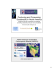### Analysis qualifying exam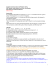### compact and resource efficient cities? synergies and trade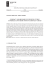### Skyline CPV36 Japanese Translation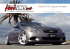### Lesson 21: Ptolemy`s Theorem### Alf van der Poorten and Continued Fractions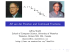### Hyperbolic manifolds, discrete groups and ergodic theory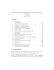### A measurement-based approach to maximum power transfer for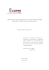### Final Four presentation board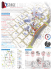### Introduction to Geometric Probability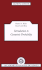### 781. - Clarkwork.com### Rick Joyner - MorningStar TV